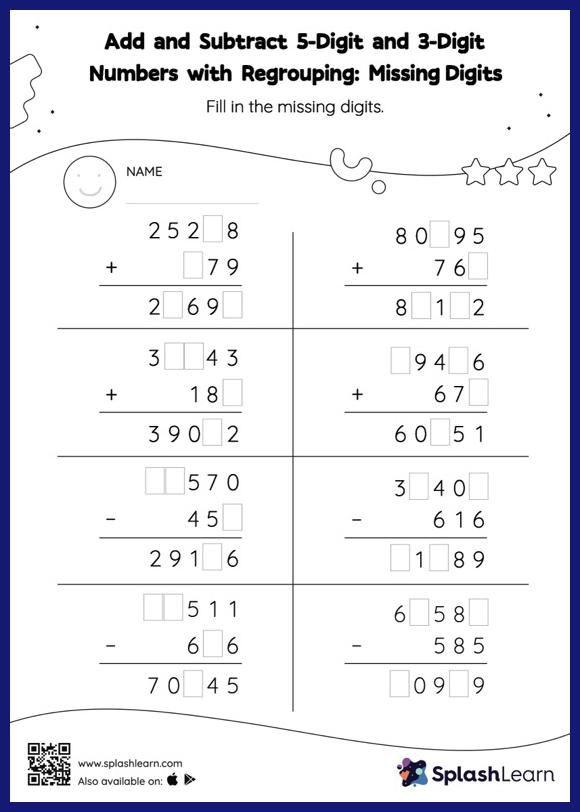# Add and Subtract 5-Digit and 3-Digit Numbers with Regrouping: Missing Digits Worksheet

Home > Add and Subtract 5-Digit and 3-Digit Numbers with Regrouping: Missing DigitsThis add and subtract 5-digit and 3-digit numbers with regrouping worksheet consists of a set of questions on addition and subtraction, which help students develop fluency. To find the missing number on this worksheet, students can utilize the connection between addition and subtraction. In add and subtract 5-digit and 3-digit numbers with regrouping worksheet, they must additionally regroup numbers to reach the solution.